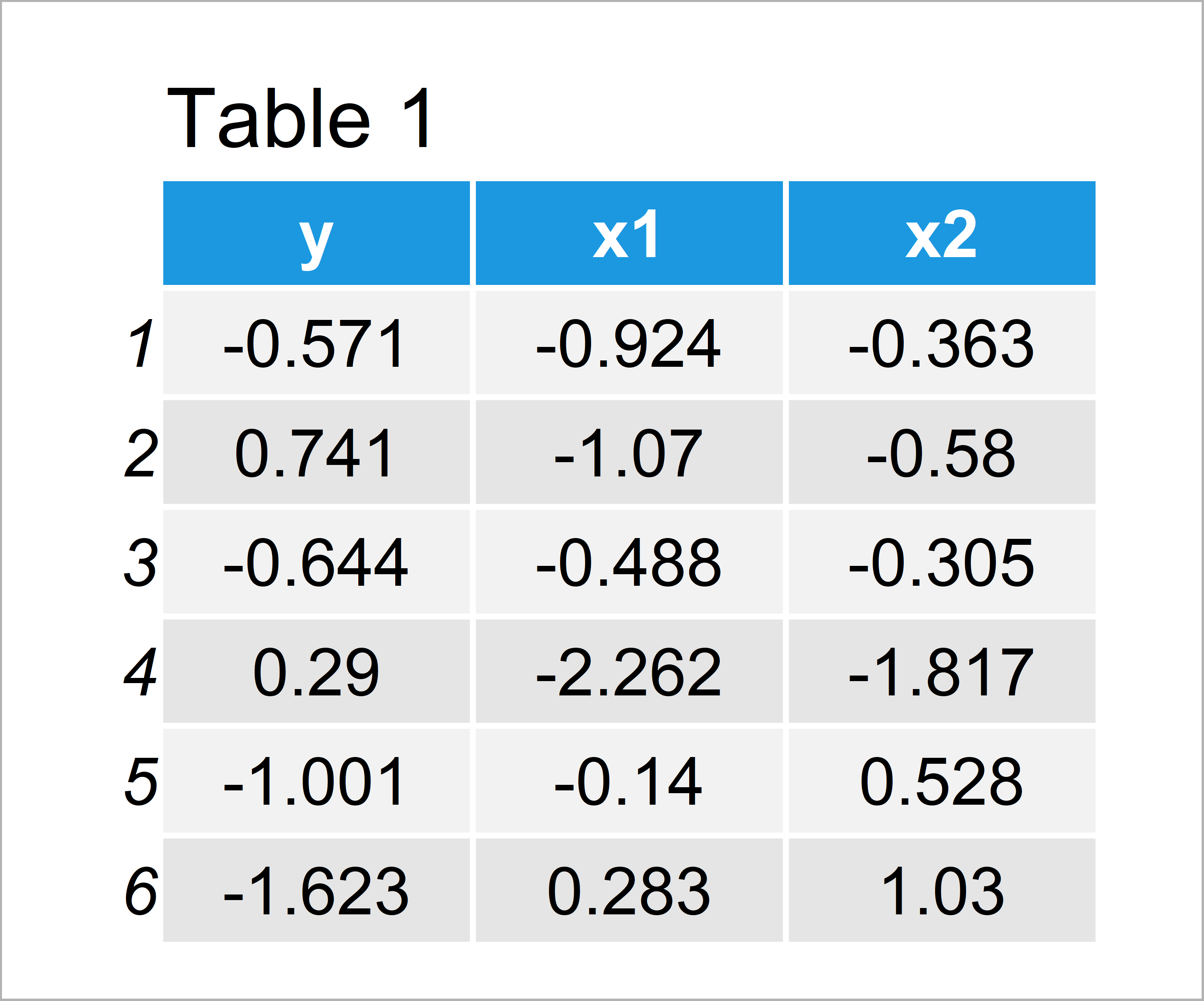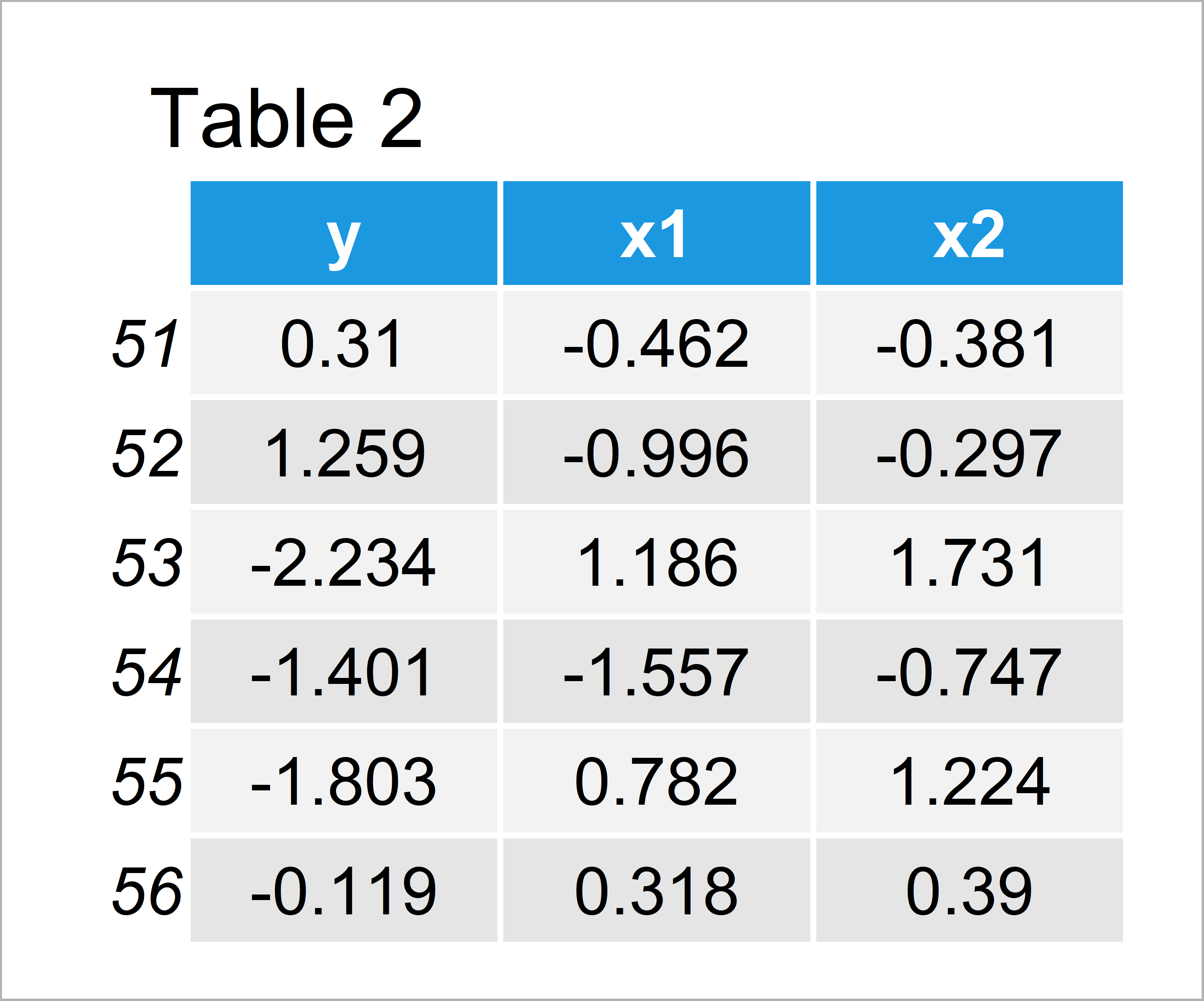# R predict Error in eval(predvars, data, env) : numeric ‘envir’ arg not of length one

This tutorial illustrates how to deal with the “Error in eval(predvars, data, env) : numeric ‘envir’ arg not of length one” in R.

Let’s get started.

## Introducing Example Data

Consider the following random example data:

```set.seed(69282)                               # Construct random example data
x1 <- rnorm(1000)
x2 <- x1 + runif(1000)
y <- 0.7 * x1 - x2 + rnorm(1000)```

Now, we can use the previously created random data to create two data frames in R:

```data_1 <- data.frame(y, x1, x2)[1:500, ]      # Create first data frameAs you can see based on Table 1, our first example data is a data frame and consists of 500 lines and three variables. We’ll use this data frame to estimate a linear model later on.

Let’s create another data frame:

```data_2 <- data.frame(y, x1, x2)[51:1000, ]    # Create second data frameIn Table 2 it is shown that we have created a second data frame that does also contain 500 rows and the same columns as the first data frame. We’ll use this data frame as basement for our predictions.

We can now estimate a linear model and create summary statistics of the first data frame using the summary and lm functions:

```my_mod <- lm(y ~ ., data_1)                   # Linear model based on first data
summary(my_mod)                               # Calculate descriptive statistics
#
# Call:
# lm(formula = y ~ ., data = data_1)
#
# Residuals:
#      Min       1Q   Median       3Q      Max
# -2.84944 -0.66255  0.00081  0.72526  2.53181
#
# Coefficients:
#             Estimate Std. Error t value Pr(>|t|)
# (Intercept) -0.07309    0.09235  -0.791 0.429054
# x1           0.55345    0.15973   3.465 0.000577 ***
# x2          -0.83167    0.15674  -5.306 1.69e-07 ***
# ---
# Signif. codes:  0 ‘***’ 0.001 ‘**’ 0.01 ‘*’ 0.05 ‘.’ 0.1 ‘ ’ 1
#
# Residual standard error: 1.004 on 497 degrees of freedom
# Multiple R-squared:  0.1148,	Adjusted R-squared:  0.1112
# F-statistic: 32.23 on 2 and 497 DF,  p-value: 6.923e-14
#```

Next, we’ll try to use this model to make predictions for our second data frame. This is the part where the problems appear!

## Error in eval(predvars, data, env) : numeric ‘envir’ arg not of length one – Why does it appear?

Let’s first discuss why the “Error in eval(predvars, data, env) : numeric ‘envir’ arg not of length one” occurs.

The reason for this error message is an incorrect specification of the data argument within the predict function.

For example, you might have specified a vector object as the data argument instead of a data frame.

In the next section of this tutorial, I’ll explain how to avoid the “Error in eval(predvars, data, env) : numeric ‘envir’ arg not of length one”.

## Error in eval(predvars, data, env) : numeric ‘envir’ arg not of length one – How to Fix

In Example 2, I’ll illustrate how to apply the predict function without getting the “Error in eval(predvars, data, env) : numeric ‘envir’ arg not of length one”.

Have a look at the following R code:

```pred_values2 <- predict(my_mod, data_2)       # Proper specification of data argument
head(pred_values2)                            # First six predicted values
#         51         52         53         54         55         56
# -0.4559808 -0.4567569 -0.2204002  0.1306103  0.4035727 -0.4010424```

As you can see based on the previous RStudio console output, we have managed to create a vector of predicted values.

## Video, Further Resources & Summary

In case you need further info on the R codes of this article, you may watch the following video of my YouTube channel. I’m explaining the examples of this page in the video:

Furthermore, you might want to read some of the related tutorials of this website. You can find some interesting articles on similar topics such as lines and coding errors below.

In summary: This tutorial has shown how to avoid the “Error in eval(predvars, data, env) : numeric ‘envir’ arg not of length one” in the R programming language. If you have additional questions, don’t hesitate to let me know in the comments.

Subscribe to the Statistics Globe Newsletter